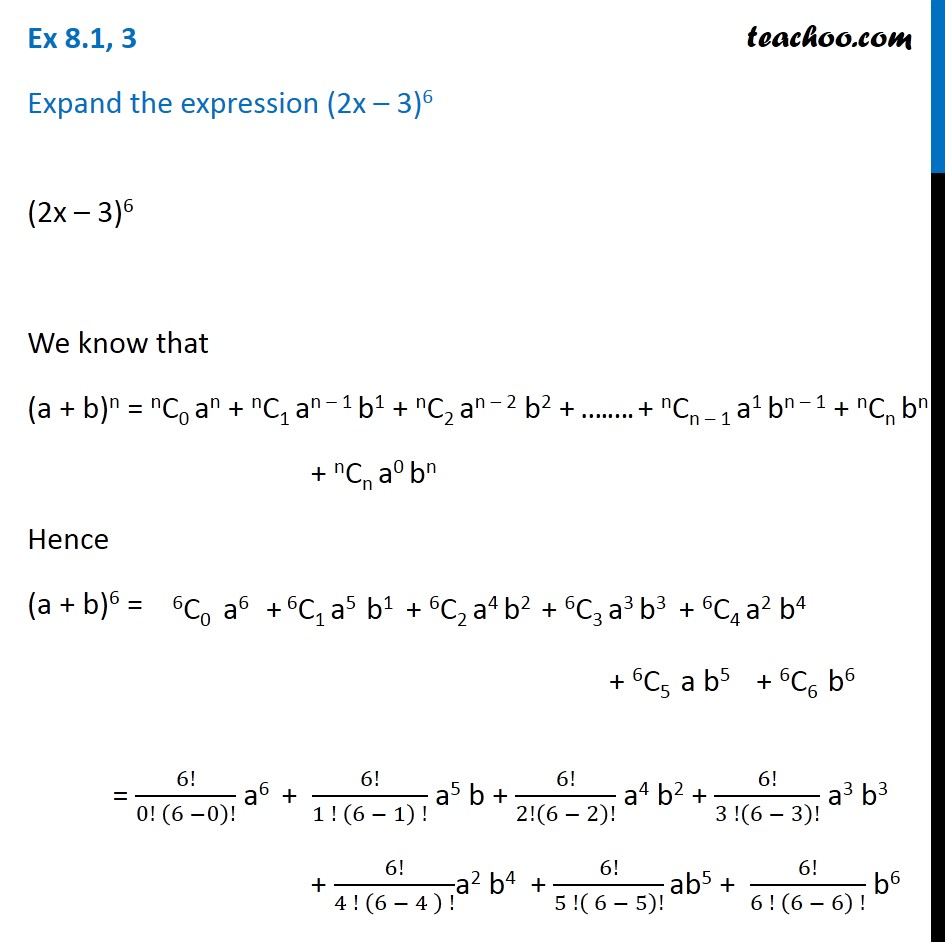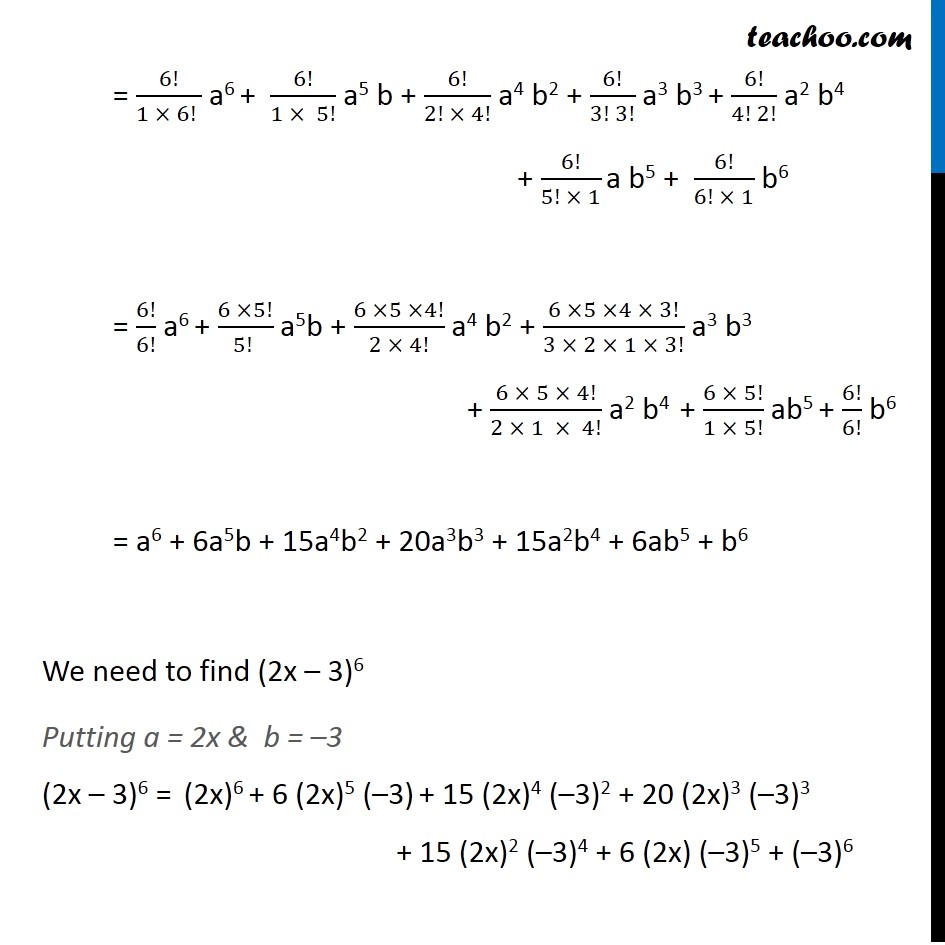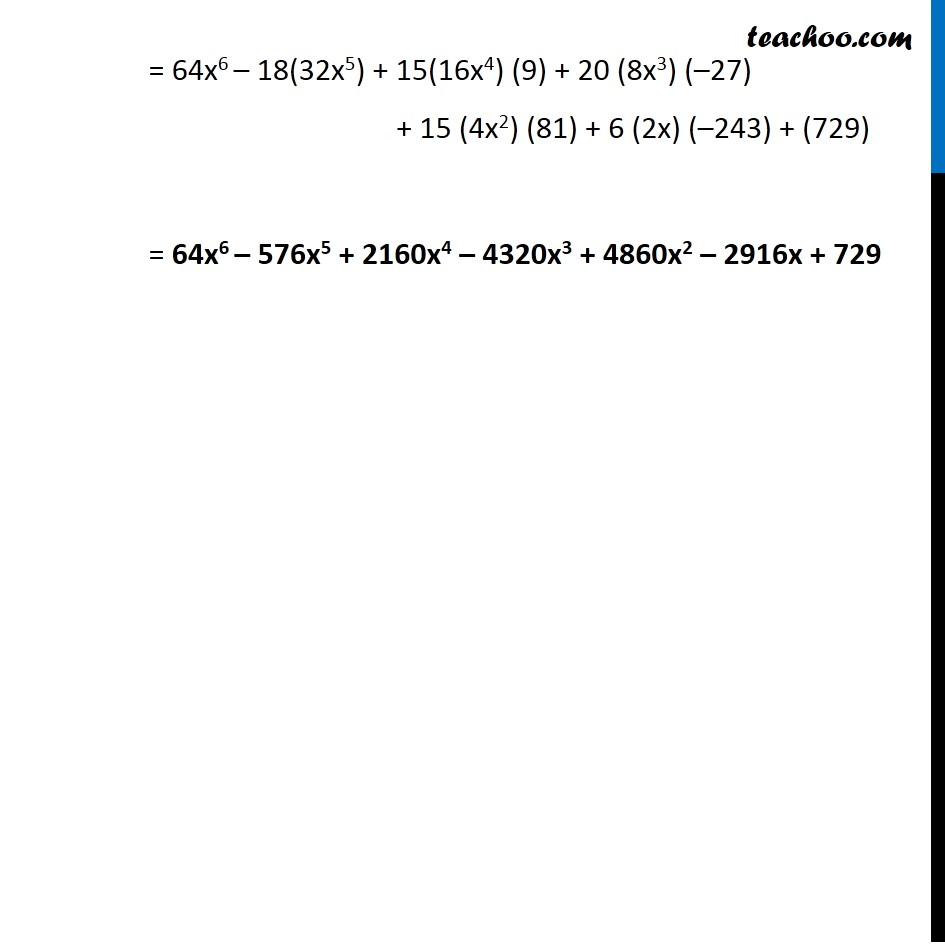Expansion

Chapter 8 Class 11 Binomial Theorem
Concept wiseIntroducing your new favourite teacher - Teachoo Black, at only ₹83 per month

### Transcript

Ex 8.1, 3 Expand the expression (2x – 3)6 (2x – 3)6 We know that (a + b)n = nC0 an + nC1 an – 1 b1 + nC2 an – 2 b2 + ….…. + nCn – 1 a1 bn – 1 + nCn bn + nCn a0 bn Hence (a + b)6 = = 6!/(0! (6 −0)!) a6 + 6!/(1 ! (6 − 1) !) a5 b + 6!/2!(6 − 2)! a4 b2 + 6!/(3 !(6 − 3)!) a3 b3 + 6!/(4 ! (6 − 4 ) !)a2 b4 + 6!/(5 !( 6 − 5)!) ab5 + 6!/(6 ! (6 − 6) !) b6 = 6!/(1 × 6! ) a6 + 6!/(1 × 5!) a5 b + 6!/(2! × 4!) a4 b2 + 6!/(3! 3!) a3 b3 + 6!/(4! 2!) a2 b4 + 6!/(5! × 1) a b5 + 6!/(6! × 1) b6 = 6!/6! a6 + (6 ×5!)/(5! ) a5b + (6 ×5 ×4!)/(2 × 4!) a4 b2 + (6 ×5 ×4 × 3!)/(3 × 2 × 1 × 3!) a3 b3 + (6 × 5 × 4!)/(2 × 1 × 4!) a2 b4 + (6 × 5!)/(1 × 5!) ab5 + 6!/6! b6 = a6 + 6a5b + 15a4b2 + 20a3b3 + 15a2b4 + 6ab5 + b6 We need to find (2x – 3)6 Putting a = 2x & b = –3 (2x – 3)6 = (2x)6 + 6 (2x)5 (–3) + 15 (2x)4 (–3)2 + 20 (2x)3 (–3)3 + 15 (2x)2 (–3)4 + 6 (2x) (–3)5 + (–3)6 = 64x6 – 18(32x5) + 15(16x4) (9) + 20 (8x3) (–27) + 15 (4x2) (81) + 6 (2x) (–243) + (729) = 64x6 – 576x5 + 2160x4 – 4320x3 + 4860x2 – 2916x + 729